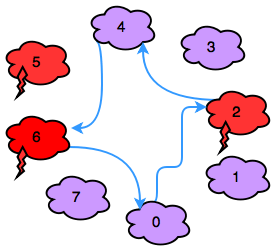# Jumping on the Clouds: Revisited Hackerrank problem solution

#### Jumping on the Clouds: Revisited Hackerrank

Aerith is playing a cloud game! In this game, there are n clouds numbered sequentially from 0 to n-1. Each cloud is either an ordinary cloud or a thundercloud.

Aerith starts out on cloud 0 with energy level E=100. She can use 1 unit of energy to make a jump of size k to cloud (i+k)%n, and she jumps until she gets back to cloud 0. If Aerith lands on a thundercloud, her energy (E) decreases by 2 additional units. The game ends when Aerith lands back on cloud 0.

Given the values of n, k, and the configuration of the clouds, can you determine the final value of E after the game ends?

Note: Recall that % refers to the modulo operation.

Input Format

The first line contains two space-separated integers, n(the number of clouds) and k(the jump distance), respectively.
The second line contains n space-separated integers describing the respective values of clouds c0,c1,…,cn-1. Each cloud is described as follows:

If ci=0, then cloud i is an ordinary cloud.
If ci=1, then cloud i is a thundercloud.

Explanation
2<=n<=25 1<=k<=n n%k=0 ci{0,1}

Output Format

Print the final value of E on a new line.

Sample Input

8 2
0 0 1 0 0 1 1 0
Sample Output

92
Explanation

In the diagram below, red clouds are thunderclouds and purple clouds are ordinary clouds:game board

Observe that our thunderclouds are the clouds numbered 2, 5, and 6. Aerith makes the following sequence of moves:

Move: 0–>2, Energy: E=100-1-2=97.
Move: 2–>4, Energy: E=97-1=96.
Move: 4–>6, Energy: E=96-1-2=93.
Move: 6–>0, Energy: E=93-1=92.
Thus, we print 92 as our answer.

##### Solution
```#include <cmath>
#include <cstdio>
#include <vector>
#include <iostream>
#include <algorithm>
using namespace std;

int main(){
int n;
int k;
int e = 101;
cin >> n >> k;
vector<int> c(n);
for(int c_i = 0;c_i < n;c_i++){
cin >> c[c_i];
}
for(int i = n/k; i >= 0; i--){
if(c[k*i] == 1){
e = e-2;
}
e = e-1;
}
cout << e;
return 0;
}```

Python

``````n,k =map(int, raw_input().split())
c = map(int, raw_input().split())
E = 100

for i in range(0,n,k):
E = E - 1 - 2*c[i]

print E
``````
``````Python 2
n, k = map(int, raw_input().split())
c = map(int, raw_input().split())
thunder_cloud = 0

for i in range(0,n,k):
thunder_cloud = thunder_cloud + c[i]

print 100 - n/k - thunder_cloud*2``````
``````n, k = map(int, raw_input().strip().split())
print 100 - sum(3**v for v in map(int, raw_input().strip().split())[::k])``````

Java

``````import java.io.*;
import java.util.*;
import java.text.*;
import java.math.*;
import java.util.regex.*;

public class Solution {

public static void main(String[] args) {
Scanner in = new Scanner(System.in);
int n = in.nextInt();
int k = in.nextInt();
int c[] = new int[n];
for(int c_i=0; c_i < n; c_i++){
c[c_i] = in.nextInt();
}

int curr = 0;
int e = 100;
curr = (curr+k)%n;
e -= 1+c[curr]*2;
while (curr != 0) {
curr = (curr+k)%n;
e -= 1+c[curr]*2;
}
System.out.println(e);
}
}
``````

### hasectic

A web developer(Front end and Back end), and DBA at csdamu.com. Currently working as Salesforce Developer @ Tech Matrix IT Consulting Private Limited. Check me @about.me/s.saifi